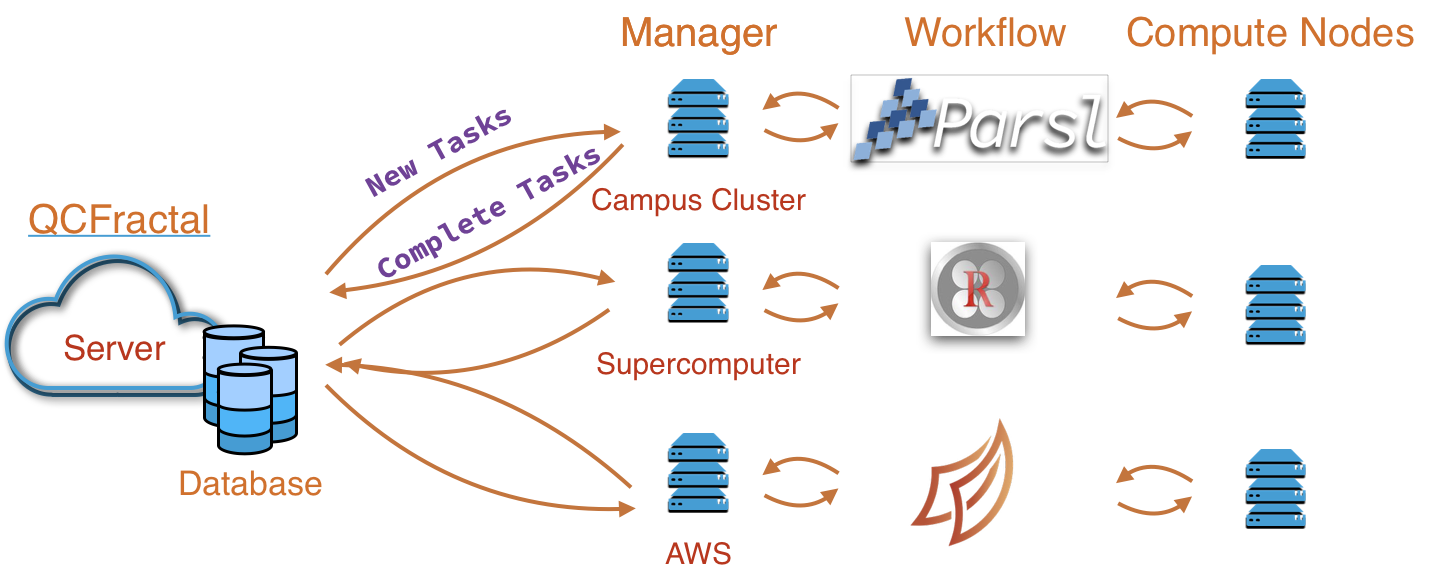You can run this notebook online in a Binder session or view it on Github.

In addition to querying existing datasets, the QCArchive software can be used to easily generate new ones. In this example, we will create a new dataset of small molecules with up to 3 heavy atoms and compute their DFT and AN1-1x energies.

For this example, we will use a demonstation “Snowflake” server which runs calculations locally in this Jupyter notebook session. In general, QCArchive can be used with thousands of distributed compute nodes at once.

:

import numpy as np
import pandas as pd
import qcportal as ptl

from qcfractal import FractalSnowflakeHandler

server = FractalSnowflakeHandler()
local_client = server.client()


Our new dataset will be called “QM3”:

:

qm3 = ptl.collections.Dataset(name="QM3", client=local_client, default_units="hartree")


## Adding molecules to a dataset¶

The following function counts heavy atoms in a molecule:

:

def count_heavy_atoms(molecule):
return len(list(filter(lambda a: a != 'H', molecule.symbols)))


The add_entry function adds a molecule to a dataset. Below, we add all molecules in QM7b with 3 or fewer heavy atoms. The save function commits these changes to the server. First, pull QM7b down from the MolSSI server:

:

client = ptl.FractalClient()
qm7b = client.get_collection("dataset", "QM7b")
qm7b_mols = qm7b.get_molecules()

:

for molecule in qm7b_mols["molecule"]:
if count_heavy_atoms(molecule) <= 3:
qm3.save()

:

'1'


We can now query the server for the molecules in our dataset:

:

qm3.get_molecules()

:

molecule
index
CH4_b3 Geometry (in Angstrom), charge = 0.0, mult...
C2H6_f3 Geometry (in Angstrom), charge = 0.0, mult...
C2H3N_a0 Geometry (in Angstrom), charge = 0.0, mult...
C2H7N_57 Geometry (in Angstrom), charge = 0.0, mult...
C2H4O_07 Geometry (in Angstrom), charge = 0.0, mult...
C2H6O_18 Geometry (in Angstrom), charge = 0.0, mult...
C2H4O_15 Geometry (in Angstrom), charge = 0.0, mult...
C2H6O_69 Geometry (in Angstrom), charge = 0.0, mult...
C2H4_96 Geometry (in Angstrom), charge = 0.0, mult...
C2H2_66 Geometry (in Angstrom), charge = 0.0, mult...
C3H6_d1 Geometry (in Angstrom), charge = 0.0, mult...
C3H8_5c Geometry (in Angstrom), charge = 0.0, mult...
C3H6_17 Geometry (in Angstrom), charge = 0.0, mult...
C3H4_11 Geometry (in Angstrom), charge = 0.0, mult...
C2H5N_1a Geometry (in Angstrom), charge = 0.0, mult...
C2H7N_5e Geometry (in Angstrom), charge = 0.0, mult...

And look at one of them:

:

qm3.get_molecules(subset="C2H6O_18")


## Running calculations¶

Our QM3 dataset now has all of its molecules, but no properties have been computed for them.

:

qm3.list_values()

:

keywords name
native driver program method basisThe compute function is used to submit calculations for every molecule in a dataset. We will compute the ωB97x/6-31g(d) energy for each molecule using the Psi4 program, and the ANI-1x energy using the TorchANI program. (Other supported programs include CFOUR, entos, GAMESS, Q-Chem, Molpro, MOPAC, NWChem, RDKit, TeraChem, and Turbomole.)

:

qm3.compute(program='psi4', method='wB97x', basis='6-31g(d)')

:

<ComputeResponse(nsubmitted=16 nexisting=0)>

:

qm3.compute(program="torchani", method="ANI1x")

:

<ComputeResponse(nsubmitted=16 nexisting=0)>


The calculations are submitted and run asynchronously.

As before, values are described with list_values and queried with get_values. Incomplete calculations show up as NaN, pandas placeholder for missing data.

:

qm3.list_values()

:

keywords name
native driver program method basis
True energy psi4 wb97x 6-31g(d) None WB97X/6-31g(d)-Psi4
torchani ani1x None None ANI1X-Torchani
:

dft_data = qm3.get_values(program='psi4')
dft_data

:

WB97X/6-31g(d)-Psi4
CH4_b3 -40.4996
C2H6_f3 -79.8015
C2H3N_a0 -132.714
C2H7N_57 -135.122
C2H4O_07 -153.746
C2H6O_18 -154.99
C2H4O_15 -153.786
C2H6O_69 -154.979
C2H4_96 -78.5573
C2H2_66 -77.2971
C3H6_d1 -117.863
C3H8_5c -119.106
C3H6_17 -117.867
C3H4_11 -116.613
C2H5N_1a -133.885
C2H7N_5e -135.13
:

ml_model_data = qm3.get_values(program='torchani')
ml_model_data

:

ANI1X-Torchani
CH4_b3 -40.4997
C2H6_f3 -79.8012
C2H3N_a0 -132.714
C2H7N_57 -135.122
C2H4O_07 -153.746
C2H6O_18 -154.99
C2H4O_15 -153.786
C2H6O_69 -154.978
C2H4_96 -78.5572
C2H2_66 -77.2973
C3H6_d1 -117.863
C3H8_5c -119.106
C3H6_17 -117.867
C3H4_11 -116.613
C2H5N_1a -133.885
C2H7N_5e -135.13

We can compare the ANI-1x predictions to the DFT values:

:

import plotly.express as px

data = pd.merge(dft_data, ml_model_data, left_index=True, right_index=True)

data["Unsigned Difference (Hartree)"] = np.abs(data["WB97X/6-31g(d)-Psi4"] - data["ANI1X-Torchani"])

fig = px.violin(data, y="Unsigned Difference (Hartree)", box=True,
title="Difference Distribution between ANI-1x and ωB97x/6-31g(d)")
fig.show()


### Other calculations you can do¶

• Dataset: Single point, gradients, and freqencies

• ReactionDataset: Reactions and interaction energies, with many-body counterpoise corrections

• OptimizationDataset: Geometry optimization

• TorsionDriveDataset: PES scans over torsion angles, for force field fitting.

• (AI)MD trajectories?

• Normal mode sampling?

### The QCArchive stack enables large-scale, multi-resource calculations¶### Ongoing data enrichment efforts¶

With the QCArchive framework, it is very easy to submit new calculations on existing datasets. In the MolSSI database, we are augmenting existing datasets with a common set of calculations at various levels of DFT:

• HF / Def2-TZVP

• LDA / Def2-TZVP

• PBE-D3M(BJ) / Def2-TZVP

• B3LYP-D3M(BJ) / Def2-TZVP

• ωB97x-D3(BJ) / Def2-TZVP

and, where feasible:

• MP2 / cc-pVTZ

• CCSD(T) / cc-pVTZ

Let us know if there are properties/calculations that you would like!

### Extras¶

[ ]:

from IPython.core.display import HTML

def print_info(dataset):
print(f"Name: {dataset.data.name}")
print()